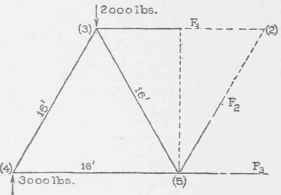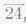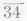38. Forces in Tension and Compression Members. As explained in "Strength of Materials" if a member is subjected to forces, any two adjacent parts of it exert forces upon each other which hold the parts together. Figs. 38 (a) and 38 (5) show how these forces act in a tension and in a compression member. F' is the force exerted on the left part by the right, and F" that exerted on the right part by the left. The two forces F' and F" are equal, and in a tension member are pulls while in a compression member they are pushes.

39. Method of Sections. To determine the stress in a member of a truss by the method explained in the foregoing (the "method of joint"), we begin at one end of the truss and draw polygons for joints from that end until we reach one of the joints to which that member is connected. If the member is near the middle of a long truss, such a method of determining the stress in it requires the construction of several polygons. It is sometimes desirable to determine the stress in a member as directly as possible without having first determined stresses in other members. A method for doing this will now be explained ; it is called the method of sections.

Fig. 39 (a) is a partial copy of Fig. 16. The line LL is intended to indicate a "section" of the truss "cutting" members 24, 34 and 36. Fig. 39 (b) and (c) represents the parts of the truss to the left and right of the section. By "part of a truss" we mean either of the two portions into which a section separates it when it cuts it completely.Fig. 39.

Since each part of the truss is at rest, all the forces acting on each part are balanced, or in equilibrium. The forces acting on each part consist of the loads and reactions applied to that part together with the forces exerted upon it by the other part. Thus the forces which hold the part in Fig. 39 (J) at rest are the 1,500-and 3,000-pound loads, the reaction 6,000 and the forces which the right part of the cut members exert upon the left parts. These latter forces are marked F1, F2' and F3'; their senses are unknown.

but each acts along the axis of the corresponding member. The forces which hold the part in Fig. 39 (c) at rest are the two 3,000-pound loads, the 1,500-pound load, the right reaction 6,000 pounds and the forces which the left parts of the cut members exert upon the right parts. These are marked F1", F2" and F3"; their senses are also unknown but each acts along the axis of the corresponding member. The forces F1" and F1', F2' and F2", and F3' and F3" are equal and opposite; they are designated differently only for convenience.

If, in the system of forces acting on either part of the truss, there are not more than three unknown forces, then those three can be computed by "applying" one of the sets of the conditions of equilibrium stated in Art 35.* In writing the equations of equilibrium for the system it is practially necessary to assume a sense for one or more of the unknown forces. We shall always assume that such forces are pulls that is, act away from the part of the truss upon which they are exerted. Then if the computed value of a force is plus, the force is really a pull and the member is in tension and if the value is minus, then the force is really a push and the member is in compression. To determine the stress in any particular member of a truss in accordance with the foregoing, "pass a section" through the truss cutting the member under consideration, and then apply as many conditions of equilibrium to all the forces acting on either part of the truss as may be necessary to determine the desired force. In passing the section, care should be taken to cut as few members as possible, and never should a section be passed so as to cut more than three, the stresses in which are unknown; neither should the three be such that they intersect in a point.Fig. 40.

*If, however, the lines of action of the three forces intersect in a point then the statement is not true.

Examples. 1. It is required to determine the amount and kind of stress in the memberof the truss represented in Fig. 39 (a) when loaded as shown.

Having determined the reactions (6,000 pounds each) we pass a section through the entire truss and cutting; LL is such a section. Considering the part of the truss to the left of the section, the forces acting upon that part are the two loads, the left reaction and the forces on the cut ends of members,and(F1', F2', and F3'). F1' can be determined most simply by writing a moment equation using (3) as the origin, for with respect to that origin the moments of F2' and F3' are zero, and hence those forces will not appear in the equation. Measuring from a large scale drawing, we find that the arm of F1' is 7 feet and that of the 3,000-pound load is 3.5 feet. Hence

(F1' X 7)+(6,000 X 14)-(1,500 X 14)- (3,000 X 3.5)= 0 or F'1 = [- (6,000 X 14) + (1,500 X 14) + (3,000 X 3.5)] / 7

= - 7,500

The minus sign means that F1' is a push and not a pull, hence the memberis under 7,500 pounds compression.

The stress in the member 24 may be computed from the part of the truss to the right of the section. Fig. 39 (c) represents that part and all the forces applied to it. To determine F1' we take moments about the intersection of F2" and F3". Measuring from a drawing we find that the arm of F1' is 7 feet, that of the load at joint (4) is 7 feet, that of the load at joint (5) is 17.5 feet, that of the load at joint (7) is 28 feet, and that of the reaction is 28 feet.